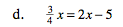### Home > MC2 > Chapter 9 > Lesson 9.1.2 > Problem9-20

9-20.
1. Simplify and solve each equation below for x. Show your work and check your answer. Homework Help ✎

1. 24 = 3x + 3

2. 2(x – 6) = x – 14

3. 3(2x – 3) = 4x – 5

4.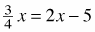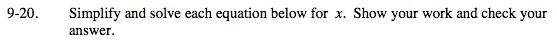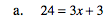Begin isolating x by subtracting 3 from both sides.

−3 + 24 = 3x + 3 − 3
21 = 3x

Divide both sides by 3.

$\frac{21}{3} = \frac{3x}{3}$

x = 7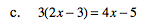Follow the methods presented in (a) and (b).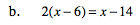Begin by distributing.

2(x) − 2(6) = x − 14
2x − 12 = x − 14